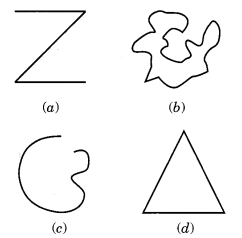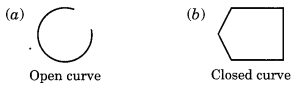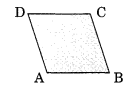# NCERT Solutions For Class 6 Maths Basic Geometrical Ideas Exercise 4.2

ncert textbook

## NCERT Solutions For Class 6 Maths Basic Geometrical Ideas Exercise 4.2

NCERT Solutions for Class 6 Maths Chapter 4 Basic Geometrical Ideas Ex 4.2

Exercise 4.2

Question 1.
Classify the following curves as (i) open or (ii) closed.Solution:
(a) Open curve
(b) Closed curve
(c) Open curve
(d) Closed curve

Question 2.
Draw rough diagrams to illustrate the following:
(a) Open curve
(b) Closed curve
Solution:Question 3.
Solution:
ABCD is the required polygon whose interior region is shaded.Question 4.
Consider the given figure and answer the questions.
(a) Is it a curve?
(b) Is it closed?Solution:
(a) Yes, it is a curve.
(b) Yes, it is closed curve.

Question 5.
Illustrate, if possible, each one of the following with a rough diagram:
(a) A closed curve that is not a polygon.
(b) An open curve made up entirely of line segments.
(c) A polygon with two sides.
Solution:
(a) Required closed curve is a circle.
(b) ABCD is an open curve made up of the line segments$\overline { AB }$,$\overline { BC }$and$\overline { CD }$.
(c) A polygon with two sides is not possible.
IMG